# Resistor Tutorial for Arduino, ESP8266 and ESP32

• Photoresistors (measure the light intensity)
• Potentiometer (a voltage divider)
• Thermistors (measure the temperature)

Also we will look deeper in the functionality of the voltage divider which gives us a basic understanding how these different kinds of resistors work.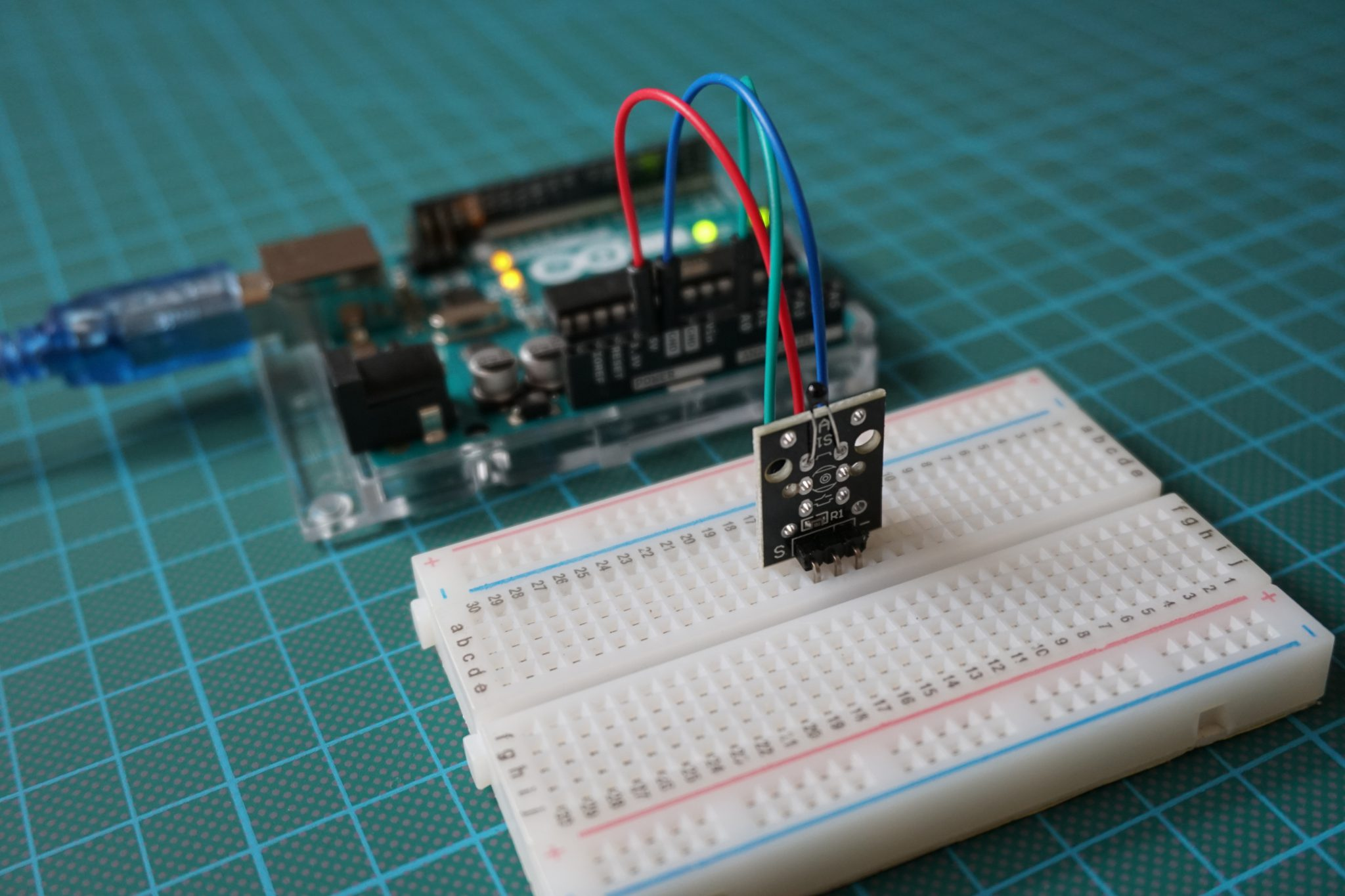## What is a Voltage Divider?

The objective of a voltage divider is to change the output voltage with the combination of 2 resistors.

One example of the use of a voltage divider is the light dependent resistor, see the second chapter in this article. Another example is a potentiometer to control the speed of a motor for example. The following example reduces the input voltage of 5V to an output voltage of 3.3V and uses a voltage divider for this task.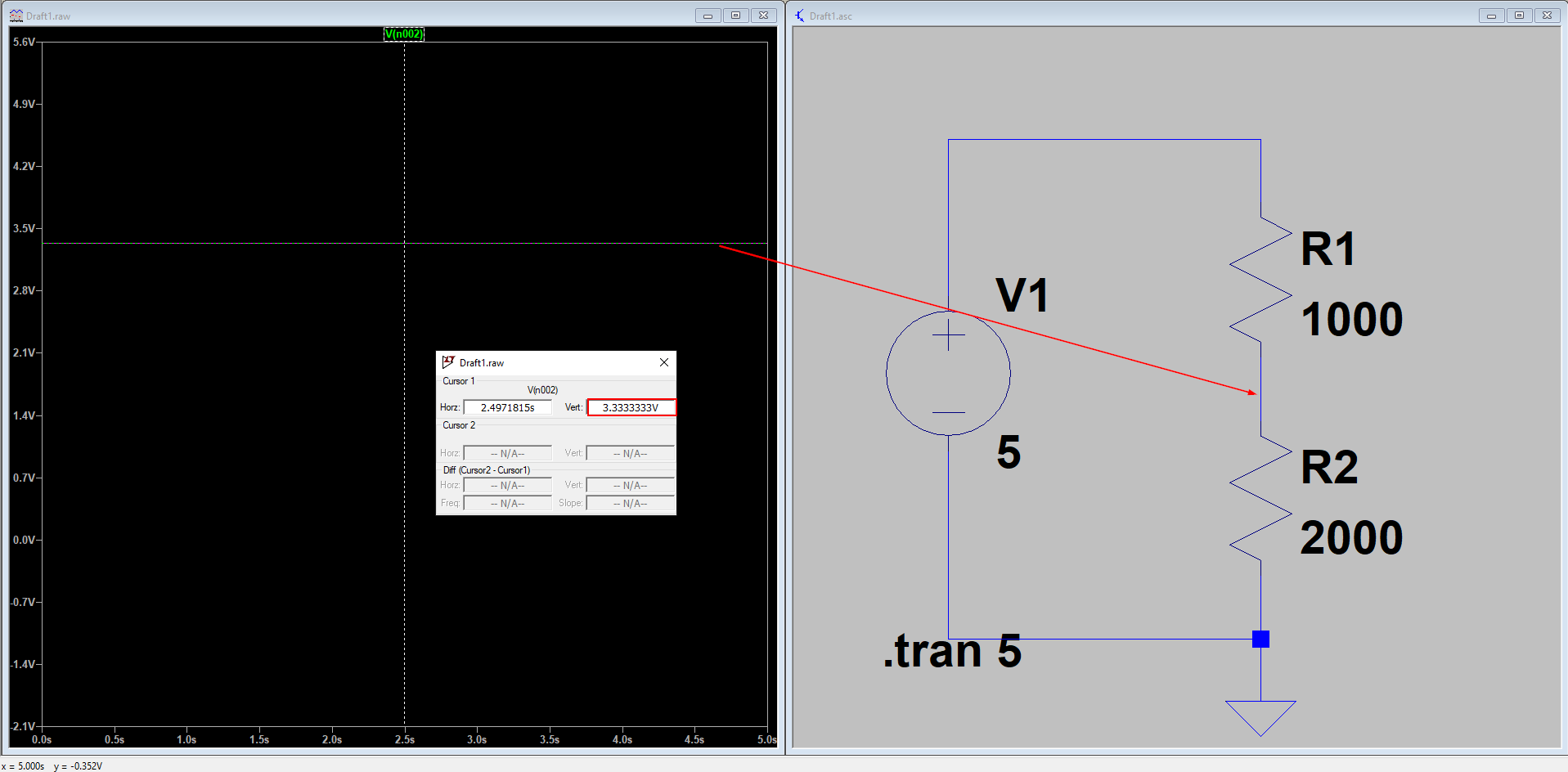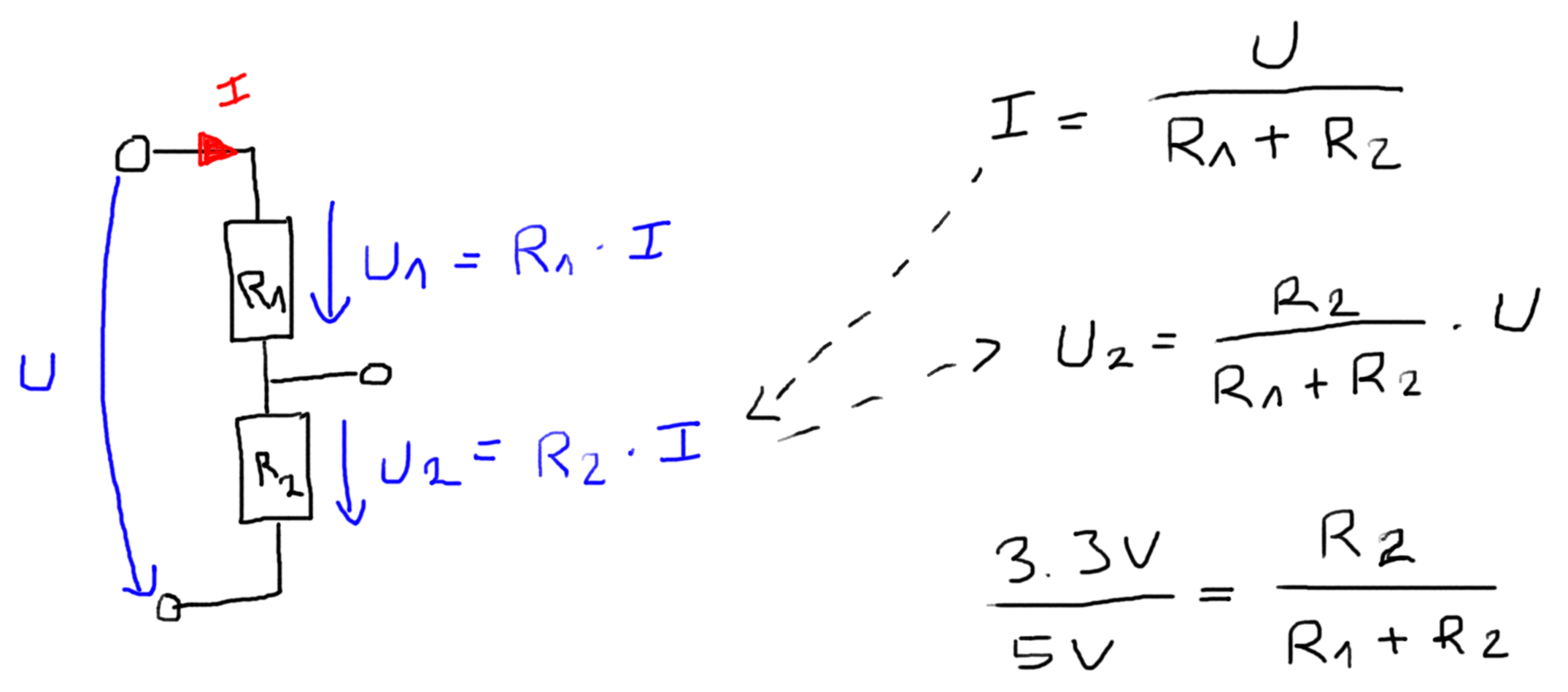The equation to calculate the output voltage from a voltage divider is given by: U2 = U* (R2/(R1+R2)). If the objective is to reduce the voltage from U=5V to U2=3.3V than multiple solutions are possible:

• R1=1kΩ and R2=2kΩ
• R1=5kΩ and R2=10kΩ.

So how do we choose the right combination? The solution is that the power to reduce the voltage and therefore the corresponding heat is not the same. The power is defined as P = U^2/(R1+R2). That’s why the combination of 5kΩ and 10kΩ resistors are producing less heat and are the preferable choice.

Keep in mind not to use a voltage divider to reduce the voltage to supply a device or load because you have to remember that an external device will result in a parallel circuit with R2. The following example show the differences in the decision to choose the resistors for the voltage divider.

Resistor
R15000Ω50Ω
R210000Ω100Ω
Resistance external device66Ω66Ω
U5V5V
R2 || Resistance external deviceR2*External device / (R2 + External device)65.57Ω39.76Ω
U2U2 = U * (R2||External device/(R1+R2||External device))0.07V2.22V
PU^2/(R1+R2||External device)4.94 mW278.52 mW

In the example you could reduce the voltage from 5V to 2.22V with the combination of R1=50Ω and R2=100Ω but your output power with 278.52 mW will likely burn the resistors.

The following table gives you an overview of all components and parts that I used for this tutorial. I get commissions for purchases made through links in this table.

Arduino Nano AmazonAliExpress
Arduino Pro Mini AmazonAliExpress
Arduino Uno AmazonAliExpress
Arduino Mega AmazonAliExpress
ESP32 ESP-WROOM-32AmazonAliExpress
ESP8266 NodeMCU AmazonAliExpress
ESP8266 WeMos D1 Mini AmazonAliExpress
Photoresistor, Potentiometer and Thermistor in Sensor Pack AmazonAliExpress

## How to Use a Photoresistor with Arduino, ESP8266 and ESP32

The photoresistor, often called light dependent resistor (LDR) or light sensor, is a resistor which
changes his resistance based on the incident light.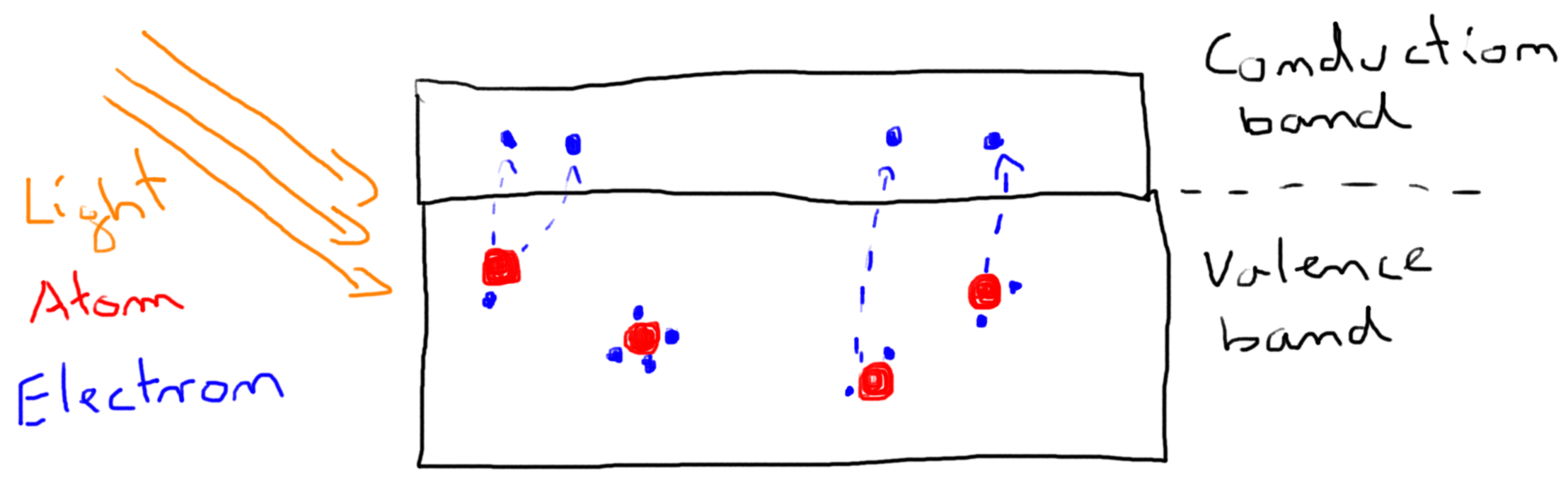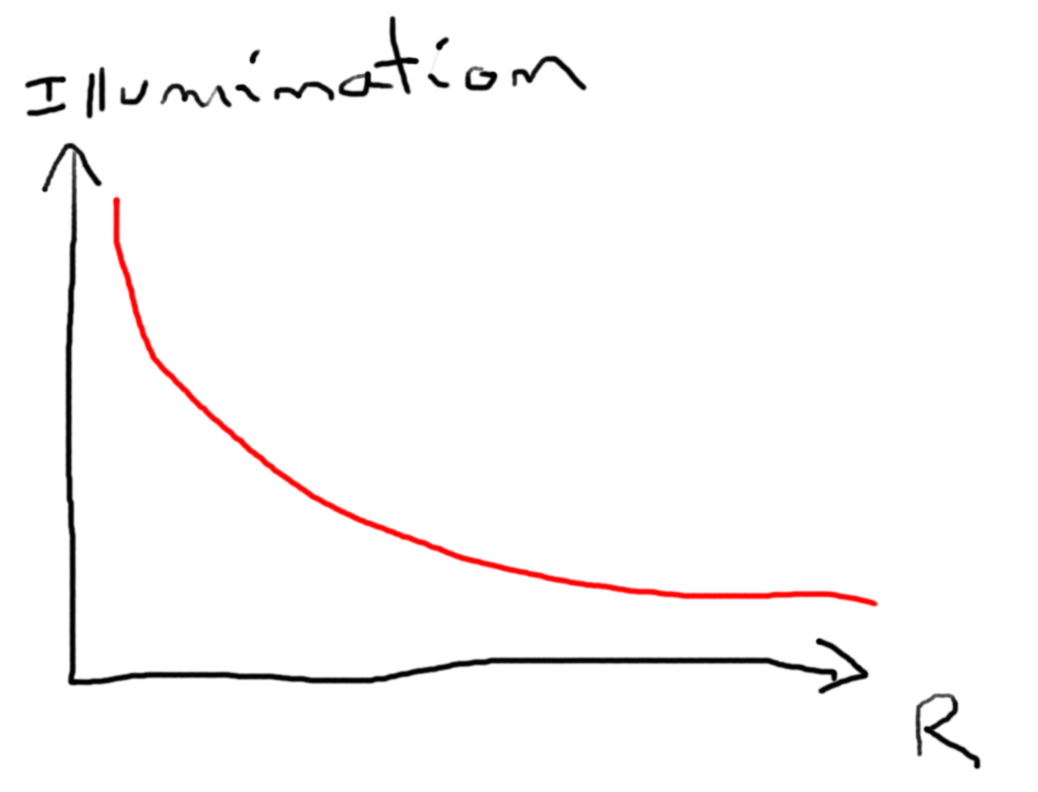The photoresistor contains electrons which are bonded to atoms in the valence band. If light falls on the photoresistor the electrons in the valence band, called valence electrons, absorb energy from the light and break the bond with the electrons. The electrons which break the bond are called free electrons and will jump into the conduction band. In the conduction band the electrons, not bonded to any atom are able to freely move from one place to another.
On the other side the atoms which previously had more electrons are now called holes. Therefore free electrons and holes are created as pairs and are carry electric current. These electric current decreases the resistance from the photoresistor.
When the energy from the light increases, more free electrons and holes are created and therefore the resistance decreases further. In summary: The resistance of the LDR decreases with increasing incident light.

To measure the incident light a voltage divider with a 4.7kΩ resistor is used.
With the analog to digital converter (ADC) the Arduino converts the reference voltage of the voltage divider to a digital value. In the following example we want to read the incident light from a light sensor and print the analog and digital values.

### Wiring between Photoresistor and Microcontroller

The following pictures show the wiring between the photoresistor, the resistor and different Arduino, ESP8266 and ESP32 microcontroller boards.

You see that the light sensor is nothing else than a resistor. Therefore you don’t have to worry about the connection sites. There is no false way to connect the light sensor.

### Code to Read the Analog Light Intensity

```#define Photoresistor A0 // for Arduino microcontroller
//#define Photoresistor A0 // for ESP8266 microcontroller
//#define Photoresistor 4 // for ESP32 microcontroller

void setup() {
Serial.begin(9600);  // set baud rate to 9600
}

void loop() {
int brightness = map(analog_value, 0, 1000, 0, 100);
Serial.println(brightness);
delay(10);
}```

The Arduino script to read the analog light intensity with a photo-resistor, starts by the definition of the connected analog pin. Because the script is written for Arduino, ESP8266 and ESP32 microcontroller, you need only one of the first three lines, depending on the microcontroller you use.

In the setup function we define the baud rate to 9600 that have to match the baud rate of the serial plotter in the Arduino IDE, where we want to visualize the analog value that represents the light intensity.

The loop functions starts by reading the voltage of the output from the voltage divider with the analogRead function and save this value in the analog_value variable. In the next step I map the analog value that can be between 0 and 1023 to a value range between 0 and 100. I use the maximum value range as 1000 because I want that analog values greater than 1000 are mapped to 100.

After the mapping of the analog input, we print the mapped value to the serial plotter and after a short delay of 10ms, the loop function starts all over.

The following video shows how the analog value is changing in the serial plotter of the Arduino IDE, depending on the light intensity that is simulated with a flashlight.Microcontroller Datasheet eBook

The 35 pages Microcontroller Datasheet Playbook contains the most useful information of 14 Arduino, ESP8266 and ESP32 microcontroller boards.

## How to Use a Potentiometer with Arduino, ESP8266 and ESP32

A potentiometer is an adjustable voltage divider and has therefore 3 connection pins. A potentiometer consists of an electrically non-conductive support on which a resistance material is applied, two terminals at the two ends of the resistive element and a movable sliding contact (also referred to as a grinder), which can divide the electrically fixed total resistance mechanically into two partial resistances corresponding to this total resistance.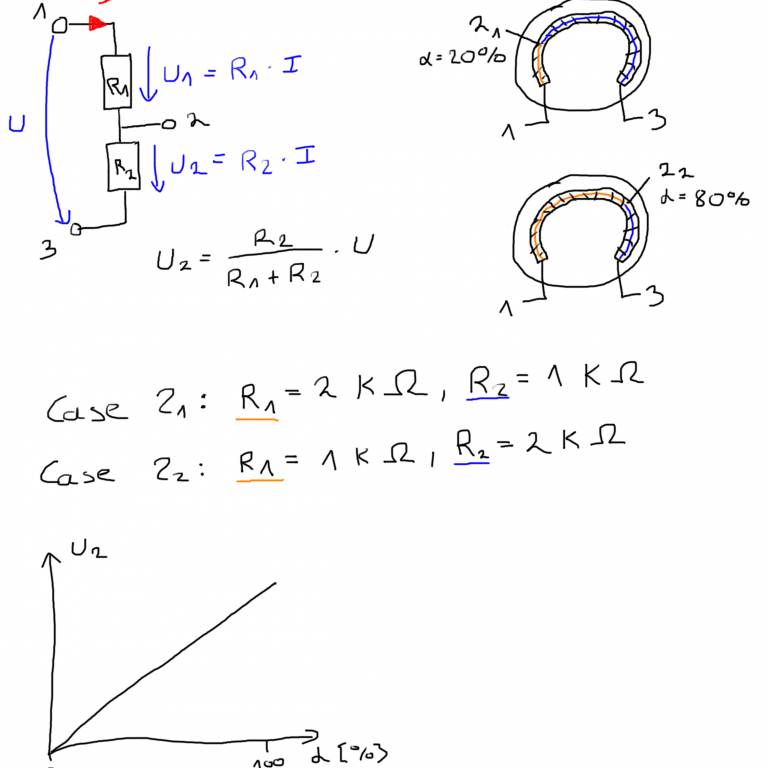### Wiring between Potentiometer and Microcontroller

The following picture show the wiring between the potentiometer and different Arduino, ESP8266 or ESP32 microcontroller boards. The output of the voltage divider is connected to the analog output of the microcontroller.

### Visualize the Output Voltage of the Potentiomter

The picture below shows how the output voltage of the voltage divider is calculated. Depending on the position of the sliding contact, the output voltage U2 is changing. I measured the output voltage in an example with my oscilloscope using R1=5kΩ and R2=10kΩ. The picture of the oscilloscope shows clearly how I changed the position of the grinder.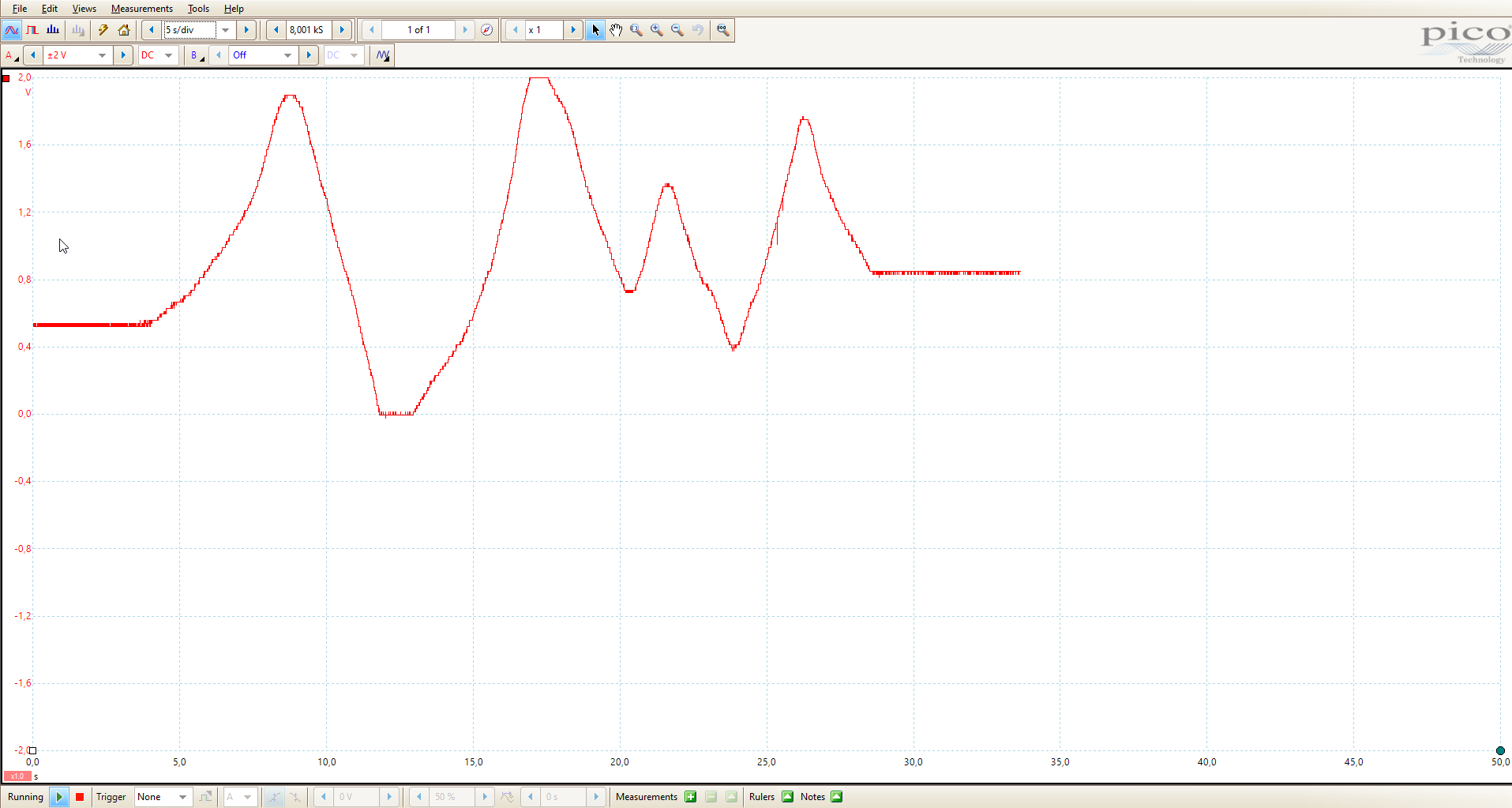Because only a few readers will have an oscilloscope, you can also use the program code from the potoresistor the measure the output voltage of the potentiometer and visualize the voltage in the serial plotter of the Arduino IDE.

### Measure the Resistance of a Potentiometer with a Multimeter

If instead of three pins only two pins are used the potentiometer acts as a variable resistor. In the following picture you see the connection between the potentiomenter and the multimeter. The following video shows the resistances measured by the multimeter in different positions of the sliding contact.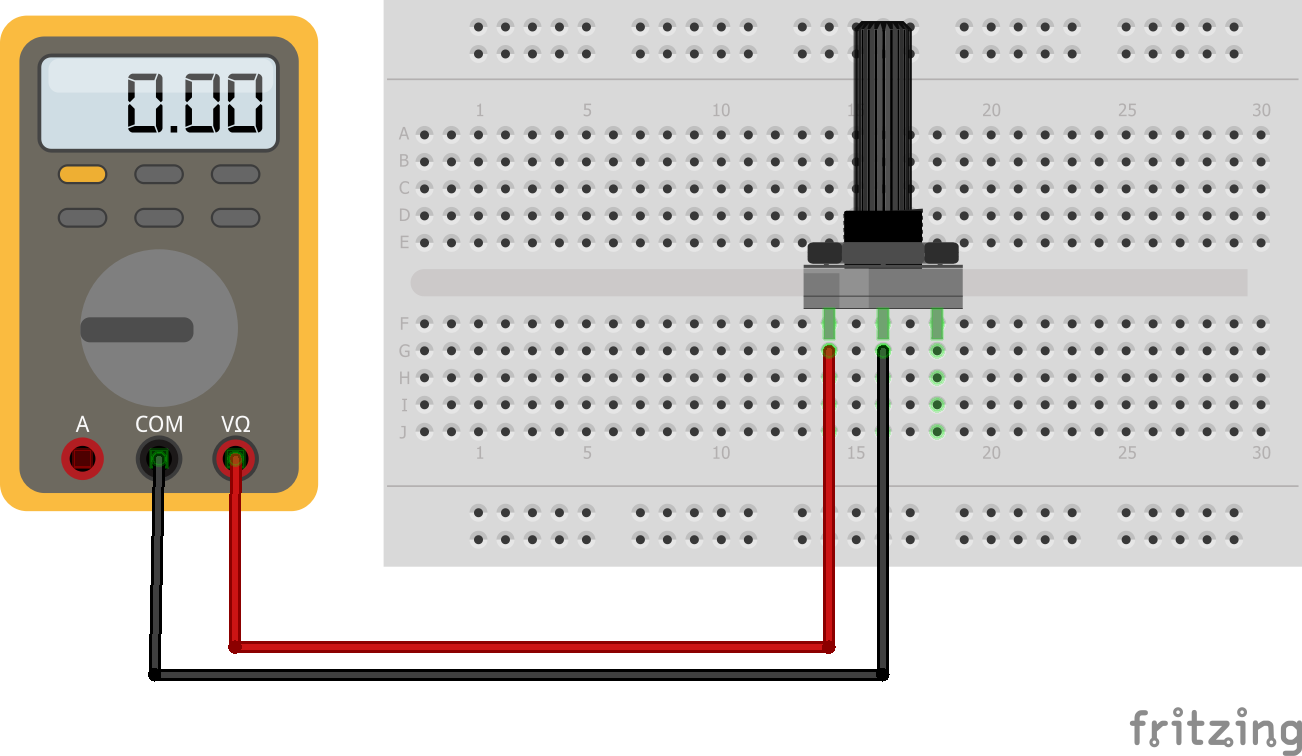## How to Use a Thermistor with Arduino, ESP8266 and ESP32

A thermistor is a resistor which changes the resistance based on the temperature. Thermistors are classified in two groups based on the behavior due to temperature changes:

• Negative Temperature Coefficient (NTC) thermistors: The resistance decreases with an increase in temperature.
• Positive Temperature Coefficient (PTC) thermistors: The resistance increases with an increase in temperature.

### Wiring between NTC Thermistor and Microcontroller

The following picture shows the wiring between the NTC thermistor and different Arduino, ESP8266 and ESP32 microcontroller. The wiring for an PTC thermistor would be the same.

The circuit is again a voltage divider, which allows us to measure the voltage drop on the thermistor. Because the resistor is directly connected to ground we have a pull-up resistor. This is important for the calculation of the resistance of the thermistor. If you do not know the difference between a pull-down and pull-up resistor, you find here an article explaining the differences in detail.

If you do not have a single thermistor but a thermistor module, where the resistor in build-in, you have to measure the resistance of the resistor with a multimeter, because we need the resistance for the calculation of the temperature in the program script. In my case thermistor module has a 10kΩ build-in.

### Code to Show the Temperature with a NTC Thermistor

Also It is possible to calculate the temperature based on the measurements of the thermistor voltage divider. Therefor we use the Steinhart equation. The following sketch code you how to measure the voltage and the temperature with a thermistor.

```#define ThermistorPin = A0; // for Arduino microcontroller
//#define ThermistorPin = A0; // for ESP8266 microcontroller
//#define ThermistorPin = 4;  // for ESP32 microcontroller

int Vo;
float R1 = 10000; // value of R1 on board
float logR2, R2, T;

//steinhart-hart coeficients for thermistor
float c1 = 0.001129148, c2 = 0.000234125, c3 = 0.0000000876741;

void setup() {
Serial.begin(9600);
}

void loop() {
R2 = R1 * (1023.0 / (float)Vo - 1.0); //calculate resistance on thermistor
logR2 = log(R2);
T = (1.0 / (c1 + c2*logR2 + c3*logR2*logR2*logR2)); // temperature in Kelvin

T = T - 273.15; //convert Kelvin to Celcius
// T = (T * 9.0)/ 5.0 + 32.0; //convert Celcius to Fahrenheit

Serial.print("Temperature: ");
Serial.print(T);
Serial.println(" C");

delay(500);
}```

The first three lines of the program code define the connected analog pin that connects the microcontroller and the thermistor. Depending on your microcontroller, you use one out of the three lines and comment the other ones or delete them.

After the definition of the analog pin, we have to define multiple variables for the Steinhart equation. Also we define the resistance, in my case 10kΩ. The Steinhart coefficients are defined for each thermistor in the datasheet.

In the setup function we only define the baud rate 9600 that has to match the baud rate of the serial monitor or serial plotter of the Arduino IDE.

The loop function stars with reading the analog value that is the output of the voltage divider. After we calculate the resistance of the thermistor, we get the temperature in Kelvin with the Steinhart equation.

Depending on your preferences you can convert the temperature from kelvin to Celsius or Fahrenheit and print the temperature to the serial output.

Conclusion

Do you have any further questions about resistors, the voltage divider, photoresistors, rotary encoder or thermistors? Use the comment section below to ask your questions.

### 2 thoughts on “Resistors Tutorial for Arduino, ESP8266 and ESP32”

1. We learned a lot